# 矮塔混凝土斜拉桥成桥索力优化分析Optimization Analysis of Bridge-Completing Cable Force for Extradosed Concrete Cable-Stayed Bridges

• 全文下载: PDF(2660KB)    PP.871-877   DOI: 10.12677/HJCE.2018.76105
• 下载量: 301  浏览量: 568   科研立项经费支持

Based on the engineering background of the Changshu Bixi Bridge (extradosed concrete ca-ble-stayed bridges (ECCB)) and by means of the influence matrix method, taking the minimum bending energy of the main girder and tower as the objective function and taking the internal force of the control section of the main girder and tower as the constraint condition, the cable force optimization analysis of the bridge was conducted. The results show that the optimized calculation of the bridge’s reasonable cable force can help to reduce the negative impact of concrete shrinkage creep and prestress loss on the bridge’s operation. The moving load has few effects on the structure state. The bridge state meets the design specification and the objective of bridge state optimization.

1. 引言

2. 工程概况与模型的建立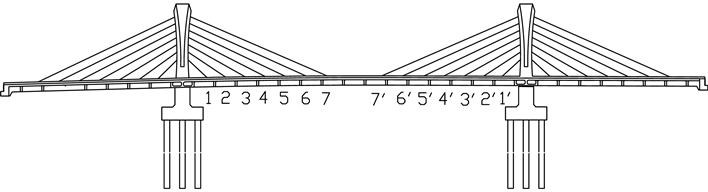Figure 1. Main bridge elevation and cable number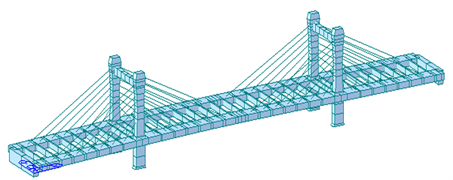Figure 2. The overall finite element model of Bixi Bridge

3. 成桥索力优化计算原理

$\left[C\right]\left\{X\right\}=\left\{D\right\}$ (1)

$U={\int }_{S}\frac{{M}^{2}\left(S\right)}{2EI}=\underset{i=1}{\overset{m}{\sum }}\frac{{L}_{i}}{4{E}_{i}{I}_{i}}\left({M}_{{L}_{i}}^{2}+{M}_{{R}_{I}}^{2}\right)$ (2)

$U={\left\{{M}_{L}\right\}}^{\text{T}}\left[B\right]{\left\{{M}_{L}\right\}}^{\text{T}}+{\left\{{M}_{R}\right\}}^{\text{T}}\left[B\right]{\left\{{M}_{R}\right\}}^{\text{T}}$ (3)

$\left\{\begin{array}{l}\left\{{M}_{L}\right\}=\left\{{M}_{{L}_{0}}\right\}+\left[{C}_{L}\right]\left\{T\right\}\\ \left\{{M}_{R}\right\}=\left\{{M}_{{R}_{0}}\right\}+\left[{C}_{R}\right]\left\{T\right\}\end{array}$ (4)

$\begin{array}{l}U={U}_{0}+{\left\{{M}_{{L}_{0}}\right\}}^{\text{T}}\left[B\right]\left[{C}_{L}\right]\left\{T\right\}+{\left\{T\right\}}^{\text{T}}{\left[{C}_{L}\right]}^{\text{T}}\left[B\right]\left\{{M}_{{L}_{0}}\right\}+{\left\{T\right\}}^{\text{T}}{\left[{C}_{L}\right]}^{\text{T}}\left[B\right]\left[{C}_{L}\right]\left\{T\right\}\\ \text{ }\text{\hspace{0.17em}}\text{\hspace{0.17em}}\text{\hspace{0.17em}}\text{+}\text{\hspace{0.17em}}{\left\{{M}_{{R}_{0}}\right\}}^{\text{T}}\left[B\right]\left[{C}_{R}\right]\left\{T\right\}+{\left\{T\right\}}^{\text{T}}{\left[{C}_{R}\right]}^{\text{T}}\left[B\right]\left\{{M}_{{R}_{0}}\right\}+{\left\{T\right\}}^{\text{T}}{\left[{C}_{R}\right]}^{\text{T}}\left[B\right]\left[{C}_{R}\right]\left\{T\right\}\end{array}$ (5)

${U}_{0}$ 为与 $\left\{T\right\}$ 无关的常数，由结构特性及其他荷载效应决定。根据变分原理，为了使结构弯曲应变能最小，则 $\frac{\partial U}{\partial {T}_{i}}=0,\left(i=1,2,3,\cdots ,n\right)$ 。n为调索的总数，即56。得到：

$\left({\left[{C}_{L}\right]}^{\text{T}}\left[B\right]\left[{C}_{L}\right]+{\left[{C}_{R}\right]}^{\text{T}}\left[B\right]\left[{C}_{R}\right]\right)\left\{T\right\}=-{\left[{C}_{R}\right]}^{\text{T}}\left[B\right]\left\{{M}_{{R}_{0}}\right\}-{\left[{C}_{L}\right]}^{\text{T}}\left[B\right]\left\{{M}_{{L}_{0}}\right\}$ (6)

4. 成桥索力优化实施流程

1) 根据设计资料，建立碧溪大桥空间有限元模型。

2) 确定各斜拉索张力荷载工况以及结构自重、预应力荷载、二期恒载等荷载工况。

3) 根据实际支承情况对有限元模型施加边界约束条件，进行一次落架成桥计算。

4) 利用“未知荷载系数”模块功能，选取弯矩应变能作为目标函数。约束条件为：索塔塔顶侧移以及主梁跨中挠度限值，索塔塔底与主梁固结处截面、主梁根部与索塔固结处截面、主梁边跨四分点截面以及主跨八分点截面弯矩限值；边墩不出现负反力。求解拉索初张力并组合荷载。

5) 判断主梁主塔的边缘应力是否超过材料容许应力，结构应力分布是否合理。否则重新调整“未知荷载系数”来调整索力。

6) 调整索力使主梁主塔的边缘应力、拉索索力相对均匀。将此荷载组合作用下的索力值作为合理成桥索力。

5. 成桥索力优化计算结果与分析Table 1. Reasonable initial tension of integral cast constructionTable 2. Reasonable bridge cable force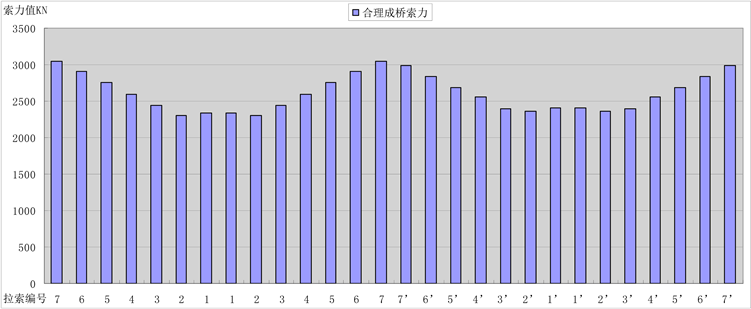Figure 3. Reasonable bridge cable force histogramFigure 4. Reasonable bridge bending moment diagram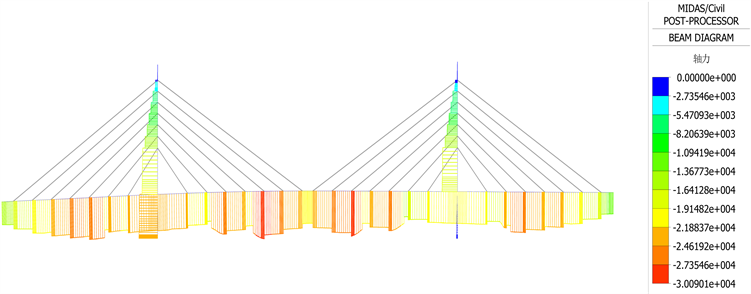Figure 5. Reasonable bridge axial force diagramFigure 6. The upper edge stress diagram of a reasonable bridge structure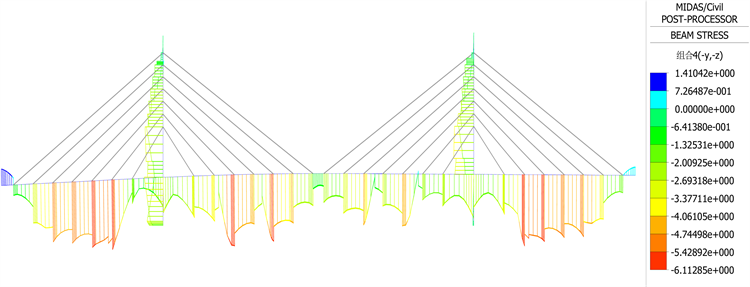Figure 7. The lower edge stress diagram of a reasonable bridge structure

6. 结语

1) 优化分析得到的大桥成桥索力比较均匀且有一定规律。

2) 在边跨和中跨跨中位置弯矩分布以小幅负弯矩为主，主梁根部位置以小幅正弯矩为主，此种内力状态有利于减小混凝土收缩徐变和预应力损失给桥梁运营带来的不利影响。

3) 对成桥状态下进行了移动车辆荷载作用分析，结果表明活载作用对混凝土矮塔斜拉桥结构状态影响较小。

NOTES

*通讯作者。

  郑一峰, 黄侨, 张连振. 部分斜拉桥结构参数分析[J]. 公路交通科技, 2006, 23(6): 60-65.  缪长青, 王义春, 黎少华. 矮塔混凝土斜拉桥成桥索力优化[J]. 东南大学学报, 2012, 42(3): 526-530.  李宇, 陈少峰. 峪道河矮塔斜拉桥拉索初张力优化[J]. 科学技术与工程, 2010, 10(17): 4312-4315.  尹梦祥. 矮塔斜拉桥施工仿真分析与索力优化研究[D]: [硕士学位论文]. 南京: 东南大学, 2017.  许慧. 关于矮塔斜拉桥的成桥索力优化[J]. 四川建材, 2018, 44(1): 121-122.  李鸿波, 祝孝成. 基于量子粒子群算法的矮塔斜拉桥索力优化[J]. 公路交通科技(应用技术版), 2017(8): 231-235.  宋涛, 宋一凡, 贺拴海, 等. 基于索梁权矩阵的矮塔斜拉桥索力优化[J]. 武汉理工大学学报(工学版), 2016, 49(2): 259-263.  Hassan, M.M. (2013) Optimization of Stay Cable in Cable-Stayed Bridges Using Finite Element, Genetic Algorithm, and B-Spline Combined Technique. Engineering Structure, 49, 643-654. https://doi.org/10.1016/j.engstruct.2012.11.036  Baldomir, A., Hernandez, S., Nieto, F., et al. (2010) Cable Optimization of a Long Span Cable Stayed Bridge in La Coruna (Spain). Advances in Engineering Software, 41, 931-938. https://doi.org/10.1016/j.advengsoft.2010.05.001